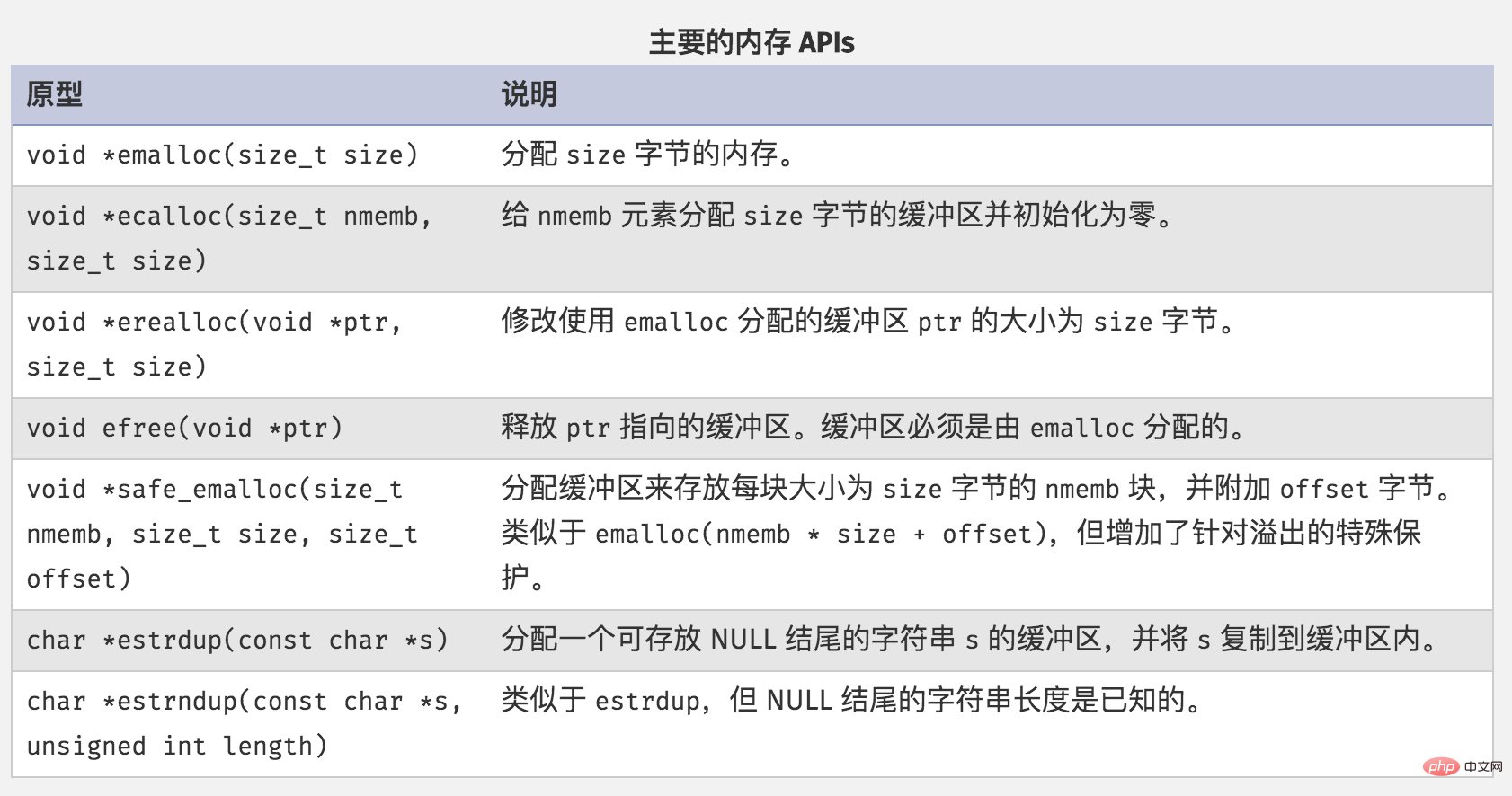# 谈谈关于PHP内存溢出的思考

1. 优化SQL语句，避免慢查询，合理的建立索引，查询指定的字段，sql优化这块在此就不展开了。
2. 查询的结果集为大对象时转数组处理，框架中一般有方法可以转，如Laravel中有toArray(),Yii2中有asArray()。
3. 对于大数组进行数据切割处理，PHP函数有array_chunk()、array_slice()。
4. 对于大型的字符串和对象，使用引用传递&。
5. 用过的变量及时unset。
6. 导出的文件格式由excel改为csv
7. ini_set(‘memory_limit’,’’)，设置程序可以使用的内存(不建议这样做)。

PHP的内存什么怎么管理的呢？ 在学C语言时，开发者是需要手动管理内存。在PHP中，Zend引擎提供为了处理请求相关数据提供了一种特殊的内存管理器。请求相关数据是只需要服务单个请求，最迟会在请求结束时释放数据。PHP5.3之前，采用引用计数的方式管理。PHP中的变量存在zval的变量容器中，变量被引用的时，引用计数+1，变量引用计数为0时，PHP将在内存中销毁这个变量。但是在引用计数循环引用时，引用计数就不会消减为0，导致内存泄漏。

PHP5.3之后做了优化，并不是每次引用计数减少都进入回收周期，只有根缓冲区满额后才开始进行垃圾回收，这样可以解决循环引用的问题，也可以将总内存泄漏保持在一个阈值之下。

 1 2 3 4 5 6 7 8 9 10 11 12 13 14 15 16 17 18 19 20 21 22 23 24 25 26 27 28 29 30 31 32 33 34 35 36 37 38 39 40 41 42 43 44 45 46 47 48 49 50 51 52 53 54 55 56 57 58 59 60 61 62 63 64 65 66 67 68 69 70 71 72 73 74 75 76 77 78 79 80 81 82 83 84 85 86 87 88 89 90 91 92 93 94 95 96 97 98 99 100 101 102 103 104 105 106 107 108 109 110 111 112 113 114 115 116 `params[``'excelSavePath'``];` `        ``foreach` `(``array_chunk``(``\$data``, 10000) ``as` `\$key` `=> ``\$value``) {` `            ``self::``\$outPutFile` `= ``''``;` `            ``\$subject`          `= !``empty``(``\$fileName``) ? ``\$fileName` `: ``'data_'``;` `            ``\$subject`          `.= ``date``(``'YmdHis'``);` `            ``if` `(``empty``(``\$value``) || ``empty``(``\$formFields``)) {` `                ``continue``;` `            ``}` `            ``self::``\$outPutFile` `= ``\$tmpPath` `. ``\$subject` `. ``\$key` `. ``'.csv'``;` `            ``if` `(!``file_exists``(self::``\$outPutFile``)) {` `                ``touch(self::``\$outPutFile``);` `            ``}` `            ``\$index`  `= ``array_keys``(``\$formFields``);` `            ``\$header` `= ``array_values``(``\$formFields``);` `            ``self::outPut(``\$header``);` `            ``foreach` `(``\$value` `as` `\$k` `=> ``\$v``) {` `                ``\$tmpData` `= [];` `                ``foreach` `(``\$index` `as` `\$item``) {` `                    ``\$tmpData``[] = isset(``\$v``[``\$item``]) ? ``\$v``[``\$item``] : ``''``;` `                ``}` `                ``self::outPut(``\$tmpData``);` `            ``}` `            ``\$fileArr``[] = self::``\$outPutFile``;` `        ``}` `        ` `        ``\$zipFile` `= ``\$tmpPath` `. ``\$fileName` `. ``date``(``'YmdHi'``) . ``'.zip'``;` `        ``\$zipRes` `= self::zipFile(``\$fileArr``, ``\$zipFile``);` `        ``return` `\$zipRes``;` `    ``}` `    ``/**` `     ``* 向文件写入数据` `     ``* @param array \$data` `     ``*/` `    ``public` `static` `function` `outPut(``\$data` `= [])` `    ``{` `        ``if` `(``is_array``(``\$data``) && !``empty``(``\$data``)) {` `            ``\$data` `= implode(``','``, ``\$data``);` `            ``file_put_contents``(self::``\$outPutFile``, iconv(``"UTF-8"``, ``"GB2312//IGNORE"``, ``\$data``) . PHP_EOL, FILE_APPEND);` `        ``}` `    ``}` `    ``/**` `     ``* 压缩文件` `     ``* @param \$sourceFile` `     ``* @param \$distFile` `     ``* @return mixed` `     ``*/` `    ``public` `static` `function` `zipFile(``\$sourceFile``, ``\$distFile``)` `    ``{` `        ``\$zip` `= ``new` `\ZipArchive();` `        ``if` `(``\$zip``->open(``\$distFile``, \ZipArchive::CREATE) !== true) {` `            ``return` `\$sourceFile``;` `        ``}` `        ``\$zip``->open(``\$distFile``, \ZipArchive::CREATE);` `        ``foreach` `(``\$sourceFile` `as` `\$file``) {` `            ``\$fileContent` `= ``file_get_contents``(``\$file``);` `            ``\$file`        `= iconv(``'utf-8'``, ``'GBK'``, ``basename``(``\$file``));` `            ``\$zip``->addFromString(``\$file``, ``\$fileContent``);` `        ``}` `        ``\$zip``->close();` `        ``return` `\$distFile``;` `    ``}` `    ` `        ``/**` `     ``* 下载文件` `     ``* @param \$filePath` `     ``* @param \$fileName` `     ``*/` `    ``public` `static` `function` `download(``\$filePath``, ``\$fileName``)` `    ``{` `        ``if` `(!``file_exists``(``\$filePath` `. ``\$fileName``)) {` `            ``header(``'HTTP/1.1 404 NOT FOUND'``);` `        ``} ``else` `{` `            ``//以只读和二进制模式打开文件` `            ``\$file` `= ``fopen``(``\$filePath` `. ``\$fileName``, ``"rb"``);` `            ``//告诉浏览器这是一个文件流格式的文件` `            ``Header(``"Content-type: application/octet-stream"``);` `            ``//请求范围的度量单位` `            ``Header(``"Accept-Ranges: bytes"``);` `            ``//Content-Length是指定包含于请求或响应中数据的字节长度` `            ``Header(``"Accept-Length: "` `. ``filesize``(``\$filePath` `. ``\$fileName``));` `            ``//用来告诉浏览器，文件是可以当做附件被下载，下载后的文件名称为\$file_name该变量的值` `            ``Header(``"Content-Disposition: attachment; filename="` `. ``\$fileName``);` `            ``//读取文件内容并直接输出到浏览器` `            ``echo` `fread``(``\$file``, ``filesize``(``\$filePath` `. ``\$fileName``));` `            ``fclose(``\$file``);` `            ``exit``();` `        ``}` `    ``}` `}`

 1 2 3 4 5 6 7 8 9 10 11 12 13 14 15 16 `\$fileName` `= ``"库存导入模板"``;` `\$stockRes` `= []; ``// 导出的数据` `\$formFields` `= [` `    ``'store_id'`  `=> ``'门店ID'``,` `    ``'storeName'` `=> ``'门店名称'``,` `    ``'sku'`       `=> ``'SKU编码'``,` `    ``'name'`      `=> ``'SKU名称'``,` `    ``'stock'`     `=> ``'库存'``,` `    ``'reason'`    `=> ``'原因'` `];` `\$fileRes`    `= ExportService::exportData(``\$fileName``, ``\$stockRes``, ``\$formFields``);` `\$tmpPath`    `= \Yii::``\$app``->params[``'excelSavePath'``]; ``// 文件路径` `\$fileName`   `= ``str_replace``(``\$tmpPath``, ``''``, ``\$fileRes``);` `// 下载文件` `ExportService::download(``\$tmpPath``, ``\$fileName``);`